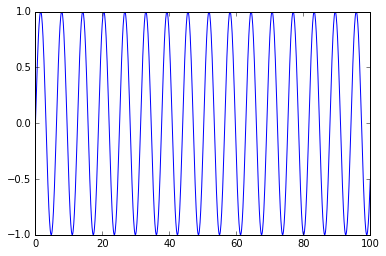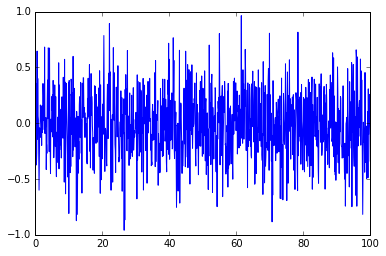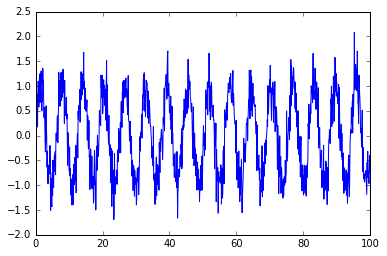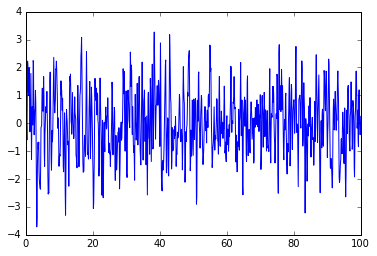# Simulating time series

We are going to simulate  time series using basic function in python.This is the first post of a series I’m going to write on signal processing, machine learning and data analysis stuff.

#### Let’s simulate a signal buried in random Gaussian noise¶

We will use also Matplolib http://matplotlib.org/ to produce plots.
Matplotlib is an excellent python library to produce any kind of plot you want.

In :
### importing the library
from __future__ import print_function
import numpy as np
import matplotlib.pyplot as plt
%matplotlib inline

In :
##number of point of the time series
nsample = 1024
## simulate a simple sinusoidal function
x1 = np.linspace(0, 100, nsample)
y=np.sin(x1)

In :
fig, ax = plt.subplots()
ax.plot(x1, y, label="Data")

Out:
[<matplotlib.lines.Line2D at 0x7f1236aa0d50>]##### Now we will add some noise.¶

We use the numpy function random.normal to add Gaussian Noise with amplitude sigma.
The Gaussian, or normal, distribution has the following probability density$\displaystyle p(x)=\frac{1}{\sqrt{2\pi\sigma^2}}e^{-\frac{\left(x-\mu\right)^2}{2\sigma^2}}$
A time series in which each sample is distributed according to a Gaussian is called Gaussian, white noise

In :
sigma = 0.3
y =sigma * np.random.normal(size=nsample)

In :
fig, ax = plt.subplots()
ax.plot(x1, y, label="Data")

Out:
[<matplotlib.lines.Line2D at 0x7f1236d39fd0>]In :
sigma = 0.3
y =np.sin(x1)+sigma * np.random.normal(size=nsample)
fig, ax = plt.subplots()
ax.plot(x1, y, label="Data")

Out:
[<matplotlib.lines.Line2D at 0x7f12368d9e90>]#### ARMA model¶

Now, let’s do something more complex and simulate the time series using an AutoRegressive Moving Average (ARMA) model.

The Notation ARMA(p,q) indicates and AR part with p poles and MA part with q zeros.$\displaystyle x[n] = \sum_{l=1}^{p} a[l]x[n-l] + \sum_{l=0}^{q} b[l]w[n-l] \ ;$

where w is a white noise with unit variance.

We can do this in many different ways and using different python library, such as scipy and its module signal, or you can write all the code from scratch.
So I’ll learn with you how to do signal processing using statsmodels.

In :
import statsmodels.api as sm
from statsmodels.tsa.arima_process import arma_generate_sample
np.random.seed(12345)

arparams = np.array([.75, -.25])
maparams = np.array([.65, .35])
arparams = np.r_[1, -arparams]
maparam = np.r_[1, maparams]

y = arma_generate_sample(arparams, maparams, nsample)
fig, ax = plt.subplots()
ax.plot(x1, y, label="Data")

Out:
[<matplotlib.lines.Line2D at 0x7f122cb63550>]#### We will see in a next posts the difference of these time series in the Frequency domain representation¶wpDiscuz